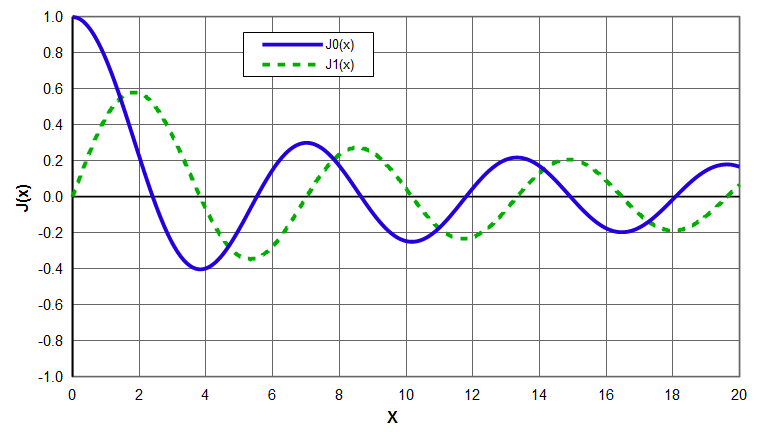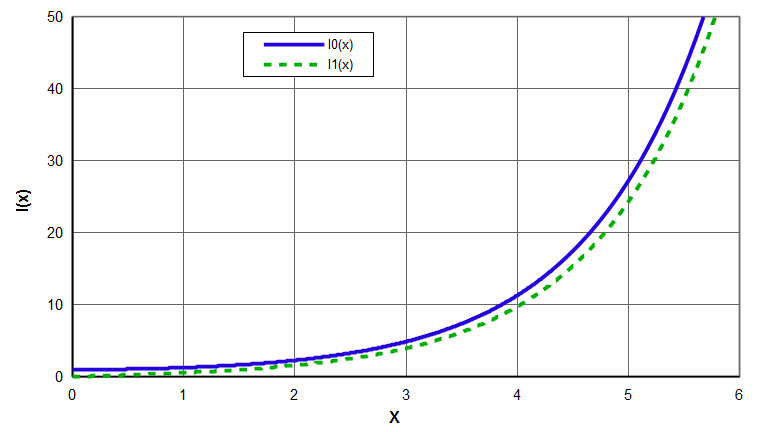# Bessel function

A function that is the solution to the differential equation —

\begin{align} \label{eq:11101a} x^2 \, \frac{d^2y}{dx^2} + x \, \frac{dy}{dx} + (x^2 - p^2) \, y = 0 \end{align}

where —

 p = a complex number (the order of the Bessel function)

Two common Bessel functions are shown in the following graph. Note that $$J_0(x)$$ resembles a decaying cosine while $$J_1(x)$$ resembles a decaying sine.Figure 1. Bessel functions - J0(x) & J1(x)

# Modified Bessel function

A function that is the solution to the differential equation —

\begin{align} \label{eq:11102a} x^2 \, \frac{d^2y}{dx^2} + x \, \frac{dy}{dx} - (x^2 + p^2) \, y = 0 \end{align}

Two common modified Bessel functions are shown in the following graph. Note that $$I_0(x)$$ resembles a hyperbolic cosine while $$I_1(x)$$ resembles a hyperbolic sine.Figure 2. Modified Bessel functions - I0(x) & I1(x)

Bessel functions and modified Bessel functions describe the flexural and radial vibration of disks and the axial amplitude of a pressure wave in an infinitely long cylinder (and many other physical phenomena).

References:
Abramowitz, pp. 355 - 434.
Kinsler, pp. 449 - 452 — includes tables for Bessel functions $$J_0(x)$$, $$J_1(x)$$, and $$J_2(x)$$ and modified Bessel functions $$I_0(x)$$, $$I_1(x)$$, and $$I_2(x)$$
Wikipedia